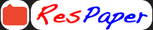Trending ▼   ResFinder# CBSE Class 10 Board Exam 2021 : Science

11 pages, 26 questions, 0 questions with responses, 0 total responses,00Vishal VinodhKumar +Fave Message ProfileTimelineUploads
 Home > vishal2006 >Formatting page ...

Formulas 1 message Lavanya kumar <vvllavanya@gmail.com> To: lavanya.vinodh <lavanya.vinodh@yahoo.co.in> 1. Real Numbers: Euclid s Division Algorithm (lemma): According to Euclid s Division Lemma if we have two positive integers a and b, then there exist unique integers q and r such that a = bq + r, where 0 r b. (Here, a = dividend, b = divisor, q = quotient and r = remainder.) 2. Polynomials: (i) (a + b)2 = a2 + 2ab + b2 (ii) (a b)2 = a2 2ab + b2 (iii) a2 b2 = (a + b) (a b) (iv) (a + b)3 = a3 + b3 + 3ab(a + b) (v) (a b)3 = a3 b3 3ab(a b) (vi) a3 + b3 = (a + b) (a2 ab + b2) (vii) a3 b3 = (a b) (a2 + ab + b2) Fri, Apr 9, 2021 at 19:45Formatting page ...Formatting page ...Formatting page ...Formatting page ...Formatting page ...Formatting page ...Formatting page ...Formatting page ...Formatting page ...Formatting page ...